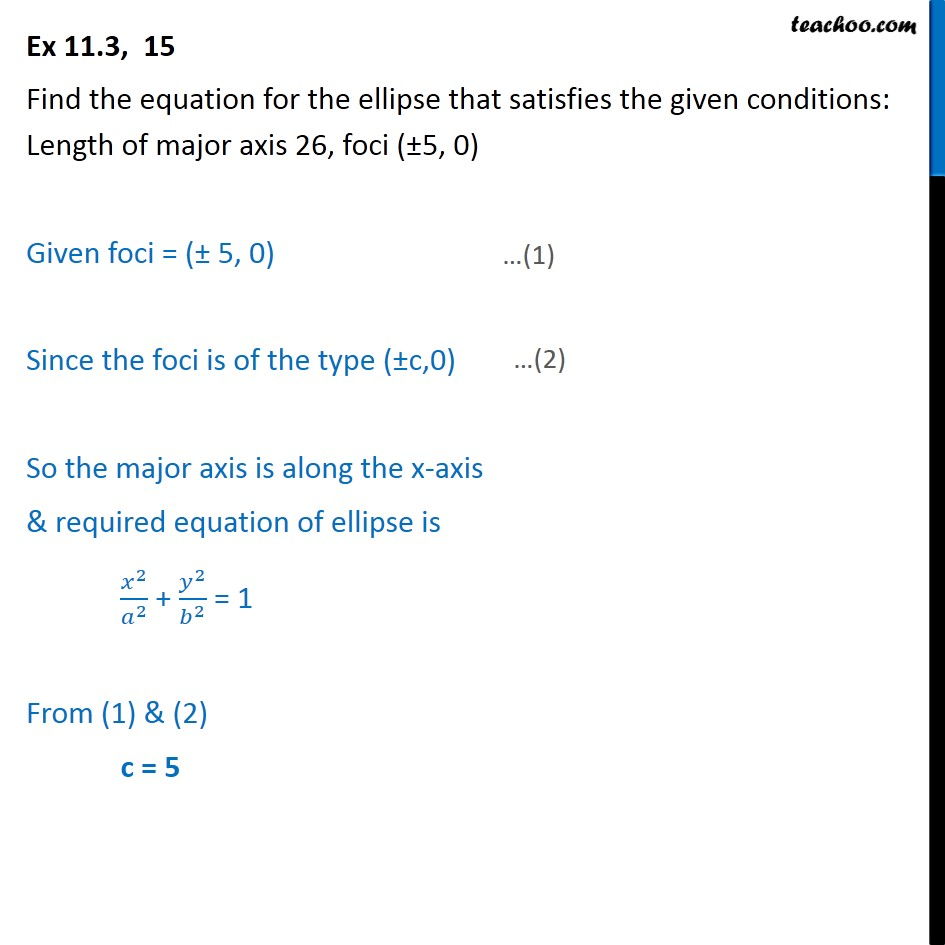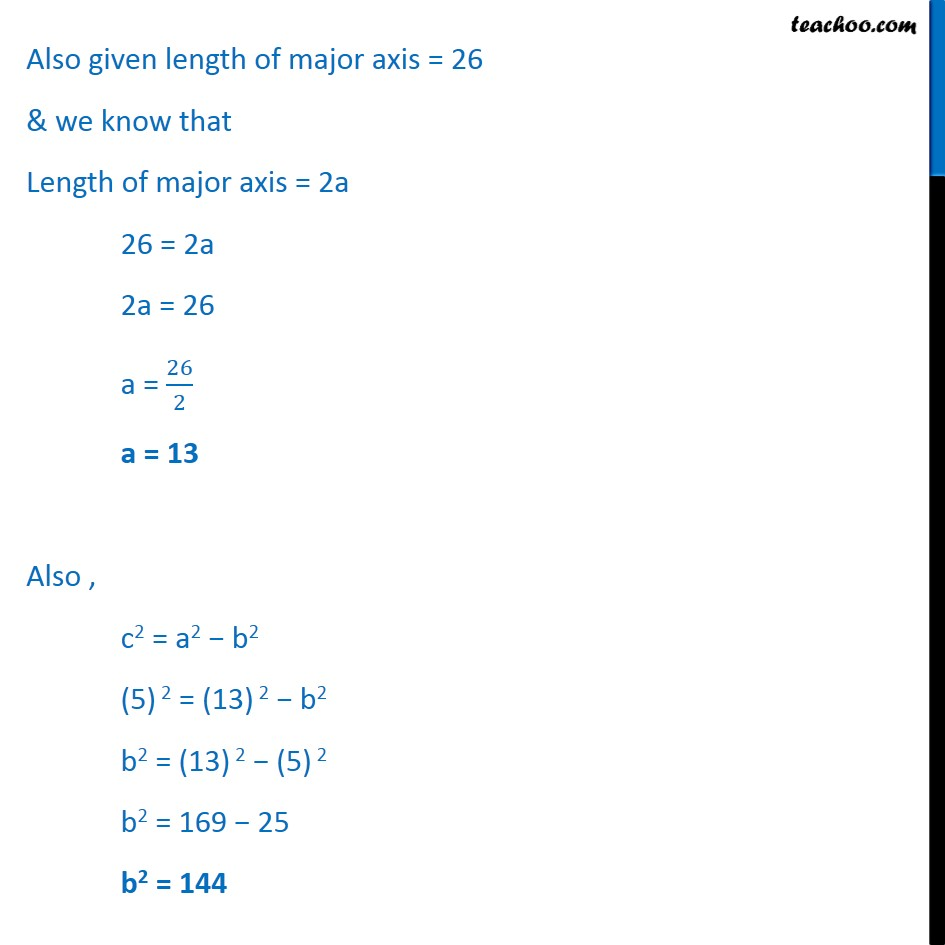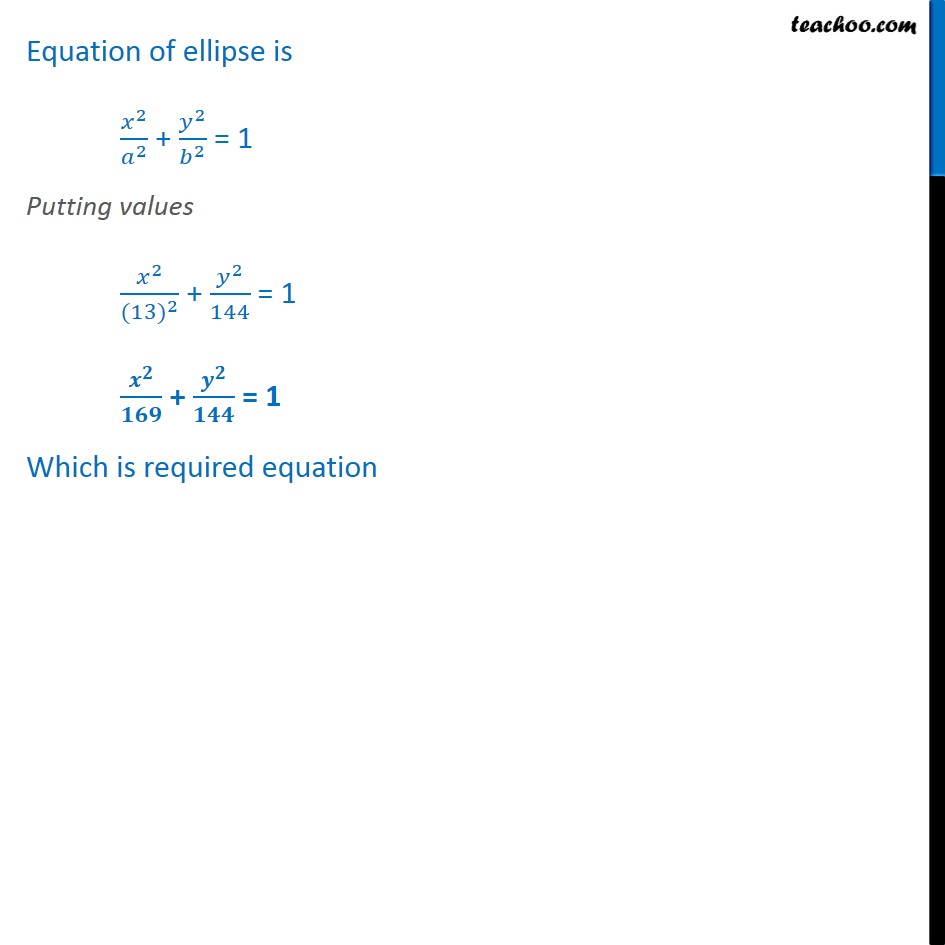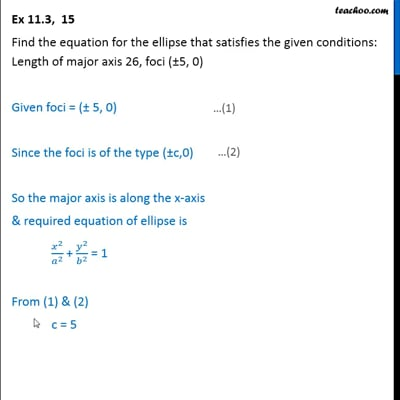Ex 11.3

Chapter 11 Class 11 Conic Sections (Term 2)
Serial order wiseThis video is only available for Teachoo black users

### Transcript

Ex 11.3, 15 Find the equation for the ellipse that satisfies the given conditions: Length of major axis 26, foci ( 5, 0) Given foci = ( 5, 0) Since the foci is of the type ( c,0) So the major axis is along the x-axis & required equation of ellipse is 2 2 + 2 2 = 1 From (1) & (2) c = 5 Also given length of major axis = 26 & we know that Length of major axis = 2a 26 = 2a 2a = 26 a = 26 2 a = 13 Also , c2 = a2 b2 (5) 2 = (13) 2 b2 b2 = (13) 2 (5) 2 b2 = 169 25 b2 = 144 Equation of ellipse is 2 2 + 2 2 = 1 Putting values 2 (13) 2 + 2 144 = 1 + = 1 Which is required equation